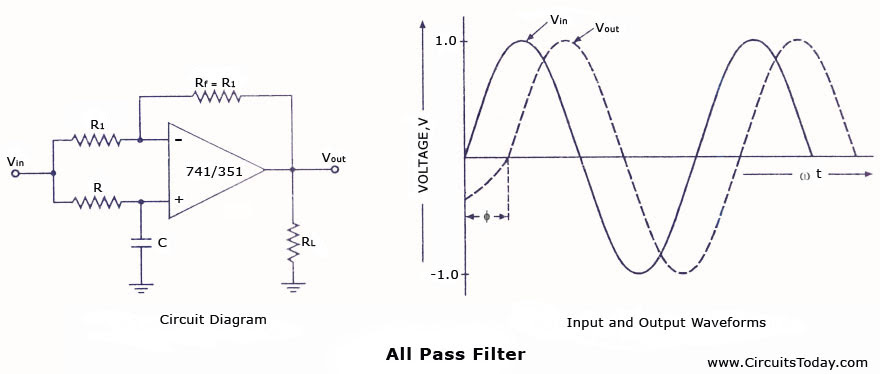Audio

[Audio][slideshow]

An all-pass filter is that which passes all frequency components of the input signal without attenuation but provides predictable phase shifts for different frequencies of the input signals. The all-pass filters are also called delay equalizers or phase correctors. An all-pass filter with the output lagging behind the input is illustrated in figure.The output voltage vout of the filter circuit shown in fig. (a) can be obtained by using the superposition theorem

vout = -vin +[ -jXC/R-jXC]2vin

Substituting -jXC = [1/j2∏fc] in the above equation, we have

vout ­= vin [-1 +( 2/ j2∏Rfc)]

or vout / vin = 1- j2∏Rfc/1+ j2∏Rfc

where / is the frequency of the input signal in Hz.

From equations given above it is obvious that the amplitude of vout / vin is unity, that is |vout | = |vin| throughout the useful frequency range and the phase shift between the input and output voltages is a function of frequency.

By interchanging the positions of R and C in the circuit shown in fig. (a), the output can be made leading the input.
These filters are most commonly used in communications. For instance, when signals are transmitted over transmission lines (such as telephone wires) from one point to an¬other point, they undergo change in phase. To compensate for such phase changes, all-pass filters are employed.

[Lights][grids]